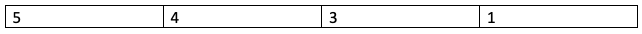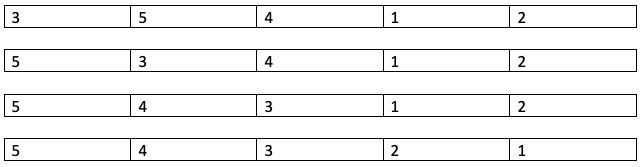# Find the Largest lexicographic array with at most K consecutive swaps

For a given array, find the largest lexicographic array which can be obtained from it after performing K consecutive swaps. Given two arrays A1 and A2, A1 is defined to be lexicographically larger than A2 if for the first i (from 0 to length of smaller array), element at index i is greater than element at index i of A2.

Note you can do less than K swaps as forcing K swaps may reduce the largeness of the result array.

We will solve this using:

• a naive approach O(N!) time
• Greedy approach O(N^2) time

## Example to understand the problem

Consider the following array:

``````A1 = [1, 33, 44, 11, 2, 5]
A2 = [1, 39, 20, 1, 0, 4]
A2 is lexicographically larger than A1 as for index 1, 39 > 33.
A3 = [2, 99, 100]
A3 is lexicographically smaller than A1 and A2 as length of A3 is smaller than length of A1 and A2.
``````

For the problem, consider this example:

``````A1 = [3, 5, 1, 4]
The lexicographically largest array is:
[5, 4, 3, 1]
With 1 consecutive swap, the largest lexicographically largest array is:
[5, 3, 1, 4]
With 2 consecutive swaps, the largest lexicographically largest array is:
[5, 3, 4, 1]
``````The lexicographically largest array for the input array is:And, the lexicographically largest array after 1 consecutive swap is:### Naive Approach `O(N!)`

A naive approach would be to generate all permutations of the array and selecting the one which satisfies the condition of K consequtive swaps. Generation of all permutations takes n! time.

The steps involved are:

1. Generate all permutations of the array.
2. Count the number of swaps.
3. If number of swaps is less than or equal to K, update lexicographically largest array.

Pseudocode:

``````Input: array[]
1. Generate all perumations of the array using some function(like next permutation)
2  Count the number of swaps:
(a)Loop through the length of the array and compare with original array.
3. If the current array is lexicographically larger than the array:
(i)Update the array lexicograph_max[]
``````

### Algorithm `O(N^2)`

We implement a greedy approach which runs as long as the value of the counter variable for swaps is less than K and the array is not lexicographically largest.

The algorithm goes as follows:

1. Loop from the 0-th index of the array to the last index of the array as long as K is non-zero.
2. For each element, find the largest element in the array which is greater than the current element and can be swapped with the current element in at most K swaps.
3. Swap the found element with the current element and update the value of K.

### Implementation

``````#include <bits/stdc++.h>
using namespace std;
int main()
{
//Obtaining length of array and number of allowed swaps
int n, k;
cin >> n >> k;
int a[n];
//Taking input of array elements
for(int i=0; i<n; i++){
cin >> a[i];
}
//Swapping elements while k is non-zero
for(int i=0; i<n-1 && k>0; i++){
//Initializing temporary index to current index
int in = i;
for(int j=i+1; j<n; j++){
//Break if max swaps are exceeded
if(k<j-i) break;
//Update maximum element index
if(a[j]>a[in]){
in = j;
}
}
//Swap the elements from in to the i-th index
for(int j=in;j>i;j--){
swap(a[j], a[j-1]);
}
//Update k after swapping in-i elements
k -= in-i;
}
//Printing lexicographically largest array
for(int i=0;i<n;i++) cout << a[i] << " ";
return 0;
}
``````

### Examples

``````//Console input
5 3
3 5 4 1 2
//Console output
5 4 3 2 1
``````

Here is the state of the array for all iterations:• In the first iteration, the first and second elements are swapped.
• In the second iteration, the second and third elements are swapped.
• In the third iteration, the fourth and fifth elements are swapped.

### Complexity

The time complexity of this algorithm is `O(N^2)`.

This is a companion discussion topic for the original entry at http://iq.opengenus.org/largest-lexicographic-array-with-at-most-k-consecutive-swaps/